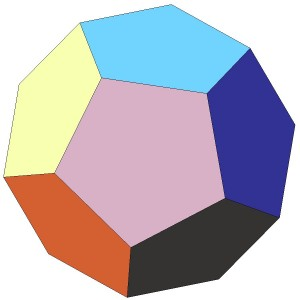# Month: September 2011

Talagrand has shown that there exists universal constants ${c,C>0}$ such that for any independent and identically distributed random variables ${X_1,\ldots,X_n}$ in the unit disk ${D}$ of ${\mathbb{C}}$, any convex and Lipschitz function ${f:D^n\rightarrow\mathbb{R}}$, and any real number ${r>0}$,

$\mathbb{P}(|f(X_1,\ldots,X_n)-\mathbb{E}f(X_1,\ldots,X_n)|\geq r) \leq C\exp(-cr^2).$

An accessible proof can be found in Ledoux’s monograph on the concentration phenomenon (chapter 4). This inequality is useful for instance in order to control the distance of a random vector to a sub-vector space of controlled dimension. In many situations, one would like a similar concentration result, beyond these restrictive assumptions. Let us consider for instance a ${n\times n}$ random matrix ${M}$ with i.i.d. entries (standard Gaussian or symmetric Bernoulli ${\pm 1}$). Here are two open questions about concentration of measure for which the Talagrand inequality is not enough as is due to the lack of one of the assumptions:

• concentration for the function ${\log|\det(M)|=\log\det\sqrt{MM^*}=\sum_{i=1}^n\log(s_i(M))}$
• concentration for the least singular value ${s_n(M)=\min_{\left\Vert x\right\Vert=1}\left\Vert Mx\right\Vert_2}$I have spent months working alone on a conjecture, ten years ago. It was at the end of my doctorate under the supervision of M. Ledoux. The problem was as follows: let ${V:\mathbb{R}\rightarrow\mathbb{R}}$ be a smooth function of the form ${V=U+B}$ with ${U}$ uniformly convex and ${B}$ bounded, such that

$\int_{\mathbb{R}}\!e^{-V(t)}\,dt=1.$

For every integer ${n\geq1}$ and real ${m}$, let ${\mu_n}$ be the product probability measure

$d\mu_n=e^{-V(x_1)-\cdots-V(x_n)}dx_1\cdots dx_n$

and let ${\mu_{n,m}}$ be the conditional exchangeable probability measure

$\mu_{n,m}=\mu(\,\cdot\,|\,x_1+\cdots+x_n=m).$

The conjecture stated that there exists a real constant ${C=C(U,B)}$ such that for every ${n\geq1}$, ${m\in\mathbb{R}}$, and every smooth function ${f:\mathbb{R}^n\rightarrow\mathbb{R}}$ in the unit sphere of ${L^2(\mu_{n,m})}$,

$\int\!f^2\log(f^2)\,d\mu_{n,m} \leq C\int\!\nabla f\cdot\nabla f\,d\mu_{n,m},$

This is a logarithmic Sobolev inequality, stronger than the Poincaré version of the conjecture which states that for every ${\mu_{n,m}}$-centered smooth ${f:\mathbb{R}^n\rightarrow\mathbb{R}}$,

$\int\!f^2\,d\mu_{n,m} \leq C\int\!\nabla f\cdot\nabla f\,d\mu_{n,m}.$

The main feature of the conjecture is the independence of ${C}$ over the constant ${m}$ and the dimension ${n}$. An important step was made by Landim, Panizo, and Yau for another right hand side, in the case where ${U}$ is quadratic and ${B,B’,B”}$ are bounded, using the martingale decomposition method of Lu-Yau or Stroock-Zegarlinski. The Poincaré version of the conjecture was almost solved by Caputo using a projection technique borrowed from a work of Carlen, Carvalho, and Loss. My modest contribution consisted in a proof of the conjecture in the case where ${U}$ is quadratic and ${B,B’,B”}$ are bounded, using the martingale technique. Later, Grunewald, Otto, Villani, and Westdickenberg provided a new technique based on a two-scales decomposition (without improving the result at that time). One can also mention some contributions by Barthe and Wolff for the Poincaré case, and by Lelièvre, among others.

Recently, Otto and his PhD student Menz have solved completely the conjecture, using the two-scales decomposition of Grunewald et al and a new one dimensional covariance estimate of the following form: for every smooth ${\nu}$-centered ${f,g:\mathbb{R}\rightarrow\mathbb{R}}$ where ${d\nu=e^{-U(t)}dt}$,

$\int\!fg\,d\nu\leq \left\Vert U”^{-1}g’\right\Vert_\infty\int\!|f’|\,d\nu.$

This new covariance estimate is a one dimensional asymmetric ${L^1-L^\infty}$ covariance version of the classical Brascamp-Lieb inequality. Some extensions of such covariance inequalities were obtained very recently by Carlen, Cordero-Erausquin, and Lieb.

The Brascamp-Lieb inequality states that if ${d\nu=e^{-H(x)}\,dx}$ is a strictly log-concave probability measure on ${\mathbb{R}^n}$, then for every ${\nu}$-centered smooth function ${f:\mathbb{R}^n\rightarrow\mathbb{R}}$,

$\int\!f^2\,d\nu \leq \int\!\nabla f\cdot (\nabla^2H)^{-1}\nabla f\,d\nu.$

The original proof of Brascamp and Lieb (1976) goes by induction on the dimension using the fact that the marginals of a log-concave probability measure are still log-concave. There is an alternative quick proof using the Witten Laplacian on closed forms. Namely, and formally, if ${f:\mathbb{R}^n\rightarrow\mathbb{R}}$ is a smooth function with zero ${\nu}$-mean, then we may write ${f=L g}$ where

$L=\Delta-\nabla H\cdot\nabla.$

A this point, we recall the (Bochner) commutation formula

$\nabla L=L\nabla – (\nabla^2 H)\nabla=\vec L\nabla \quad\mbox{where}\quad \vec L=L-\nabla^2H$

The gradient of a smooth function is a closed ${1}$-form and ${\vec L}$ acts on such forms (Witten Laplacian on forms). Taking ${\nabla g=\vec{L}^{-1}\nabla f}$ and using integration by parts,

$\int\!f^2\,d\nu =\int\!f Lg\,d\nu =-\int\!\nabla f\cdot \nabla g\,d\nu =-\int\!\nabla f \cdot \vec{L}^{-1}\nabla f\,d\nu.$

Now, as quadratic forms, since ${-L\geq0}$ we get ${-\vec L^{-1}\leq (\nabla^2 H)^{-1}}$.

This was explored by Helffer and Sjöstrand. It was also known by Hörmander (according to Cordero-Erausquin). This approach, efficient for the variance, fails miserably for the covariance.

A recent talk by Villani provides an overview on this conjecture, its recent solution, and its relation with Ginzburg-Landau dynamics and hydrodynamical limits in statistical mechanics.

Last Updated on 2014-06-17There are two kinds of convex analysis that I know a bit, corresponding to rather distinct scientific communities, taking their roots in the works of Minkowski, Carathéodory, Krein, Fenchel, Tucker, Milman I, and many others. Both domains concern finite dimensional spaces. The first one is more concerned with optimization algorithms in fixed dimension (possibly large nowadays), while the second is related to Banach spaces and involves asymptotic geometric analysis in which the dimension is often high. Both domains are connected to geometry, to probability theory, and to statistics. Here are some of the classical books (not all of them!):

Last Updated on 2014-08-19The fresh GeMeCoD ANR Project has now a collaborative web site powered by dokuwiki. The use of Dokuwiki was suggested by Laurent Marciniszyn. Wiki software are very useful for collaborative projects. I have successfully used during the past three years the wiki software MoinMoin for the former EVOL ANR Project. I also tried by curiosity MediaWiki, which is far more rich and complex than dokuwiki or MoinMoin. Like MoinMoin, dokuwiki uses a text file backend instead of a relational database such as MySQL. Such a simple design suits perfectly the needs of small projects. Dokuwiki is written in PHP while MoinMoin is written in Python. There are numerous other free wikis available, see for instance the Wikipedia page on wiki software.

You may see the dokuwiki software in action by browsing the short introduction on the Fourier-Entropy-Influence conjecture of Friedgut and Kalai that I have created recently on the GeMeCoD wiki. The mathematics are rendered with the excellent MathJax.

Syntax · Style · .